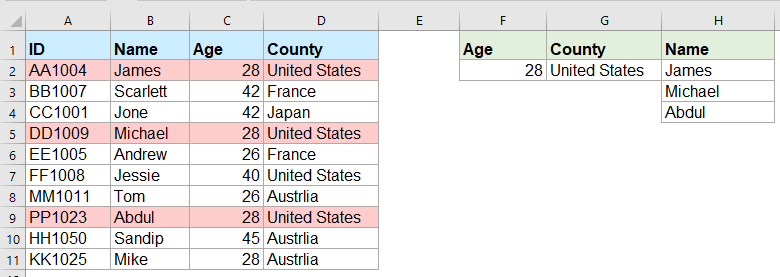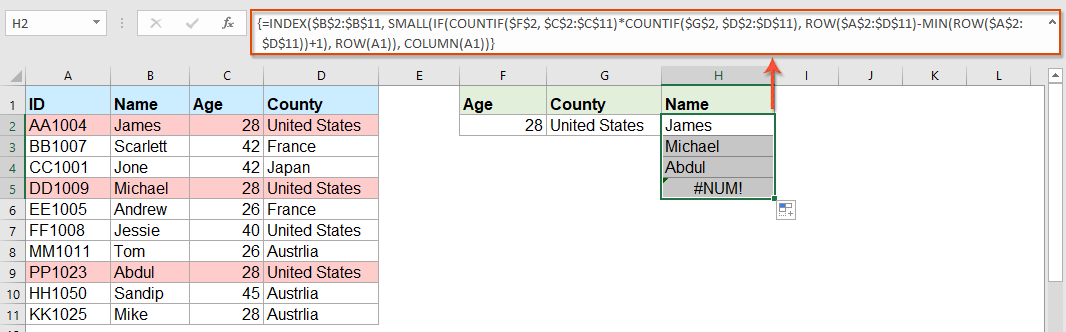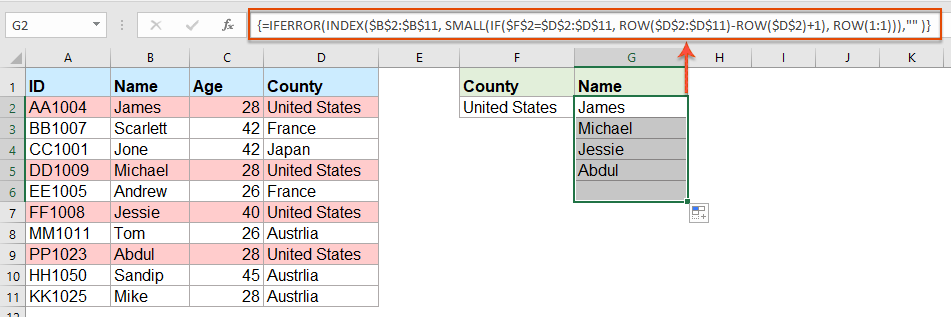Note: The other languages of the website are Google-translated. Back to English

## How to return multiple matching values based on one or multiple criteria in Excel?

Normally, lookup a specific value and return the matching item is easy for most of us by using the VLOOKUP function. But, have you ever tried to return multiple matching values based on one or more criteria as following screenshot shown? In this article, I will introduce some formulas for solving this complex task in Excel.Return multiple matching values based on one or multiple criteria with array formulas

#### Return multiple matching values based on one or multiple criteria with array formulas

For example, I want to extract all names whose age is 28 and come from United States, please apply the following formula:

1. Copy or enter the below formula into a blank cell where you want to locate the result:

=INDEX(\$B\$2:\$B\$11, SMALL(IF(COUNTIF(\$F\$2, \$C\$2:\$C\$11)*COUNTIF(\$G\$2, \$D\$2:\$D\$11), ROW(\$A\$2:\$D\$11)-MIN(ROW(\$A\$2:\$D\$11))+1), ROW(A1)), COLUMN(A1))

Note: In the above formula, B2:B11 is the column that the matching value is returned from; F2, C2:C11 are the first condition and the column data which contains the first condition; G2, D2:D11 are the second condition and the column data which contains this condition, please change them to your need.

2. Then, press Ctrl + Shift + Enter keys to get the first matching result, and then select the first formula cell and drag the fill handle down to the cells until error value is displayed, now, all matching values are returned as below screenshot shown:Tips: If you just need to return all the matching values based on one condition, please apply the below array formula:

=IFERROR(INDEX(\$B\$2:\$B\$11, SMALL(IF(\$F\$2=\$D\$2:\$D\$11, ROW(\$D\$2:\$D\$11)-ROW(\$D\$2)+1), ROW(1:1))),"" )#### More relative articles:

• Return Multiple Lookup Values In One Comma Separated Cell
• In Excel, we can apply the VLOOKUP function to return the first matched value from a table cells, but, sometimes, we need to extract all matching values and then separated by a specific delimiter, such as comma, dash, etc… into a single cell as following screenshot shown. How could we get and return multiple lookup values in one comma separated cell in Excel?
• Vlookup And Return Multiple Matching Values At Once In Google Sheet
• The normal Vlookup function in Google sheet can help you to find and return the first matching value based on a given data. But, sometimes, you may need to vlookup and return all matching values as following screenshot shown. Do you have any good and easy ways to solve this task in Google sheet?
• Vlookup And Return Multiple Values From Drop Down List
• In Excel, how could you vlookup and return multiple corresponding values from a drop down list, which means when you choose one item from the drop down list, all of its relative values are displayed at once as following screenshot shown. This article, I will introduce the solution step by step.
• Vlookup And Return Multiple Values Vertically In Excel
• Normally, you can use the Vlookup function to get the first corresponding value, but, sometimes, you want to return all matching records based on a specific criterion. This article, I will talk about how to vlookup and return all matching values vertically, horizontally or into one single cell.
• Vlookup And Return Matching Data Between Two Values In Excel
• In Excel, we can apply the normal Vlookup function to get the corresponding value based on a given data. But, sometimes, we want to vlookup and return the matching value between two values as the following screenshot shown, how could you deal with this task in Excel?

## The Best Office Productivity Tools

### Kutools for Excel Solves Most of Your Problems, and Increases Your Productivity by 80%

• Super Formula Bar (easily edit multiple lines of text and formula); Reading Layout (easily read and edit large numbers of cells); Paste to Filtered Range...
• Merge Cells/Rows/Columns and Keeping Data; Split Cells Content; Combine Duplicate Rows and Sum/Average... Prevent Duplicate Cells; Compare Ranges...
• Select Duplicate or Unique Rows; Select Blank Rows (all cells are empty); Super Find and Fuzzy Find in Many Workbooks; Random Select...
• Exact Copy Multiple Cells without changing formula reference; Auto Create References to Multiple Sheets; Insert Bullets, Check Boxes and more...
• Favorite and Quickly Insert Formulas, Ranges, Charts and Pictures; Encrypt Cells with password; Create Mailing List and send emails...
• Extract Text, Add Text, Remove by Position, Remove Space; Create and Print Paging Subtotals; Convert Between Cells Content and Comments...
• Super Filter (save and apply filter schemes to other sheets); Advanced Sort by month/week/day, frequency and more; Special Filter by bold, italic...
• Combine Workbooks and WorkSheets; Merge Tables based on key columns; Split Data into Multiple Sheets; Batch Convert xls, xlsx and PDF...
• Pivot Table Grouping by week number, day of week and more... Show Unlocked, Locked Cells by different colors; Highlight Cells That Have Formula/Name...### Office Tab - brings tabbed interface to Office, and make your work much easier

• Enable tabbed editing and reading in Word, Excel, PowerPoint, Publisher, Access, Visio and Project.
• Open and create multiple documents in new tabs of the same window, rather than in new windows.
• Increases your productivity by 50%, and reduces hundreds of mouse clicks for you every day!No ratings yet. Be the first to rate!
This comment was minimized by the moderator on the site
I tried this exact same formula; copied 100%. The only thing I changed was the data being matched and returned. When I use this formula Excel says "You've entered too many arguments for this function).=INDEX('2020 Volume Report'!\$B\$3:\$B\$100,SMALL(IF(COUNTIF(\$A\$1,'2020 Volume Report'!\$A\$3:\$A\$100)*COUNTIF(\$A\$3,'2020 Volume Report'!\$D\$3:\$D\$100),ROW('2020 Volume Report'!\$A\$3:\$G\$100)-MIN(ROW('2020 Volume Report'!\$A\$3:\$G\$100))+1,"0"),ROW(A1),COLUMN(A1))
This comment was minimized by the moderator on the site
Hi, Could you give your data and formula error as a screesnhot here?
This comment was minimized by the moderator on the site
Hello, How I can use it for Horizontal condition.
This comment was minimized by the moderator on the site
What is the "0" after the +1 in the formula? That isn't in the example one.
This comment was minimized by the moderator on the site
Hi I had tried same formula. am getting result but when give CSE it doesn't providing any multiple responses
This comment was minimized by the moderator on the site
<p>
</p>
This comment was minimized by the moderator on the site
Regarding Return Multiple Matching Values Based On One Or Multiple Criteria With Array Formulas: Why is it that if I have the data anywhere else except starting in A1 that it doesnt work even though I update all cell references in the formula?
This comment was minimized by the moderator on the site
In the first example, what change to the formula would be needed to return everyone who was less than 28 years old?
This comment was minimized by the moderator on the site
Hi,

I was wondering if it at all possible to enter a 2nd criteria but from the same range as the 1st criteria,

For example with the used example above i would like to search for the names of people from both America and France So cell F3 would have France, Scarlett & Andrew would also populate in the list in Column G

Thank you for assistance in advance.
This comment was minimized by the moderator on the site
Hello Nick,

Glad to help. If you want to get the names of people from both America and France, I advise you to use our formula twice to get the result. Please see the screenshot, In F2 and G2 are values "United States" and "France". Apply formula =IFERROR(INDEX(\$B\$2:\$B\$11, SMALL(IF(\$F\$2=\$D\$2:\$D\$11, ROW(\$D\$2:\$D\$11)-ROW(\$D\$2)+1), ROW(1:1))),"" ) to get the results for America. And apply formula =IFERROR(INDEX(\$B\$2:\$B\$11, SMALL(IF(\$G\$2=\$D\$2:\$D\$11, ROW(\$D\$2:\$D\$11)-ROW(\$D\$2)+1), ROW(1:1))),"" ) to get the results for France. It's simple. Please have a try.

Sincerely,
Mandy
This comment was minimized by the moderator on the site
When I use the second formula and drag down, nothing appears. The formula result (fx) says it should be returning something but it is blank. How do I correct this?
This comment was minimized by the moderator on the site
Hello Alysia,

Glad to help. I tried the second formula in the article and drag the formula down, the rest of results were returned. I think there may be two reasons for your problem. First, maybe you forget to press Ctrl + Shift + Enter keys to enter the formula. Second, the matching result is only one, so no other results are not returned. Please have a check.

Sincerely,
Mandy
This comment was minimized by the moderator on the site
hello,
ive tried using the formula and it either generates a value of 0 or the image attached
This comment was minimized by the moderator on the site
Hello, Milku
Your screenshot showed WPS software of MAC version, so I am not sure whether our formula is available.
I uploaded an Excel file to here, you can try to see if it can calculate correctly in you environment.
Thank you!
This comment was minimized by the moderator on the site
Hello,
what would be needed to expand the first formula in the following case:
Some IDs are Blank (e.g. cell A5 is blank) and I would like an additional condition outputting lines only when the IDs are not blank. (So the output should then be James and Abdul.
Thanks!
This comment was minimized by the moderator on the site
Hello, Jo,
``=INDEX(\$B\$2:\$B\$11, SMALL(IF(COUNTIF(\$F\$2, \$C\$2:\$C\$11)*COUNTIF(\$G\$2, \$D\$2:\$D\$11)*(\$A\$2:\$A\$11<>0), ROW(\$A\$2:\$D\$11)-MIN(ROW(\$A\$2:\$D\$11))+1), ROW(A1)), COLUMN(A1))``

This comment was minimized by the moderator on the site
Hi,

if in cell H1 i write "Name" and wanted to link that with the formula, how would that work?
Then I could write "ID" in cell H1 and would automatically get as a result: AA1004; DD1009; PP1023 (for the first formula)

This comment was minimized by the moderator on the site
Hello, Marie
Sorry, i can't get the point of your first problem, could you explain your problem more clearly and detailedly? Or you can insert a screenshot here to describe your problem.
As for the second question, you just need to change the cell reference as this:
``=INDEX(\$A\$2:\$A\$11, SMALL(IF(COUNTIF(\$F\$2, \$C\$2:\$C\$11)*COUNTIF(\$G\$2, \$D\$2:\$D\$11), ROW(\$A\$2:\$D\$11)-MIN(ROW(\$A\$2:\$D\$11))+1), ROW(A1)), COLUMN(A1))``

Remeber to press Ctrl + Shift + Enter keys together.
This comment was minimized by the moderator on the site
Heyi, thank you for the formula. It worked for "fixed" values / text as criterias. However, one of the criterias i'm trying to use is a condition (values <>0 ), but does not work the described formula. Do you guys know what should i change to adapt the formula so I can have a condition as one of the criterias, please?

Best,

João
This comment was minimized by the moderator on the site
Hello, Marcus
https://www.extendoffice.com/documents/excel/6393-excel-vlookup-function.html#b3-2
There are some detailed explanations of this task. You just need to change the criteira to your own.
Thank you!
This comment was minimized by the moderator on the site
Hi,

First, thank you for sharing!

Can you please provide a solution to the case below:

I have 3 columns (A: Containing reference information, B: Containing information to be searched, C: Search result)

Image url is provided below

https://ibb.co/VHCd09K

Column A-------------------------Column B------------Column C
File Name-------------------------Name----------------File Name, Document Name, Element Name, Name
Changed Element-----------------Element--------------Changed Element, Element Name, Element ID
Column Location
Document Name
Element Name
Name
Category
Warranty
Slope
Element ID

What I need is to search in column A for any partial match with cell B2 (Name) or B3 (Element) and get the result in one cell,

This comment was minimized by the moderator on the site
``````Public Function ConcatPartLookUp(rngInput As Range, rngSource As Range, Optional strDelimiter As String, Optional blCaseSensitive)
Dim rng As Range
If strDelimiter = "" Then strDelimiter = "，"
If IsMissing(blCaseSensitive) Then
blCaseSensitive = False
Else
blCaseSensitive = True
End If
For Each rng In rngSource
If blCaseSensitive Then
If InStr(1, rng.Value, rngInput.Value, vbBinaryCompare) > 0 Then ConcatPartLookUp = ConcatPartLookUp & strDelimiter & rng.Value
Else
If InStr(1, rng.Value, rngInput.Value, vbTextCompare) > 0 Then ConcatPartLookUp = ConcatPartLookUp & strDelimiter & rng.Value
End If
Next
If Len(ConcatPartLookUp) > 0 Then ConcatPartLookUp = Mid(ConcatPartLookUp, 2, Len(ConcatPartLookUp))
End Function
``````

After copying and pasting this code, and then use this formula:=ConcatPartLookUp(B2,\$A\$2:\$A\$8) to get the result you need.
This comment was minimized by the moderator on the site
Hi,

Thanks for posting these examples.
I'm trying to implement this in my own sheet, but don't get it to work (maybe because I'm using an europe version of excel)?

I want to get the dates of the days that I had my shifts or that I have worked 'some' (>0) hours for a client.

So in I3 is the name and in J3 the month. K3 and L3 are the shifts (1 is worked) and hours (don't know how to set this, should be more than zero)

My expected results are in:
Shifts: I7 and I8
hours: J7

So I worked more than 0 hours for 'person 2' in oktober on 3-10-2022
had shifts for person 2 on '10-10-2022' and 28-10-2022

When I add '=INDEX(\$B\$2:\$B\$11, SMALL(IF(COUNTIF(\$F\$2, \$C\$2:\$C\$11)*COUNTIF(\$G\$2, \$D\$2:\$D\$11), ROW(\$A\$2:\$D\$11)-MIN(ROW(\$A\$2:\$D\$11))+1), ROW(A1)), COLUMN(A1))' in my excel sheet, it doesn't allow the comma between the different parts of the formula.
So I need to change them to ';'.
But when I try it it always says: '#NAME?'

So can someone help me with this?

Kind regards,

Bas
This comment was minimized by the moderator on the site
Hi, if there are duplicate values (e.g. two adams), how do i make sure that it only returns 1 adam and not 2?
This comment was minimized by the moderator on the site
Hello, Bobby,
To extract only unique matching values, you should apply the below formula:
After pasting the formula, please press Ctrl + Shift + Enter keys together to get the correct result.
=IFERROR(INDEX(\$B\$2:\$B\$5, MATCH(0, COUNTIF(H1:\$H\$1, \$B\$2:\$B\$5)+IF(\$D\$2:\$D\$5<>\$G\$2, 1, 0)+IF(\$C\$2:\$C\$5<>\$F\$2, 1, 0), 0)), "")